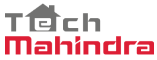New update is available. Click here to update.

# N-th Fibonacci Number

Medium0/80
40 mins
70 %470 upvotes+36 more

## Problem Statement

#### Since the answer can be very large, return the answer modulo 10^9 +7.

##### Fibonacci number is calculated using the following formula:
``````F(n) = F(n-1) + F(n-2),
Where, F(1) = F(2) = 1.
``````
##### For Example:
``````For ‘N’ = 5, the output will be 5.
``````
Detailed explanation ( Input/output format, Notes, Images )
##### Constraints:
``````1 <= T <= 10
1 <= N <= 10^5

Time Limit: 1 sec.
``````
##### Sample Input 1:
``````2
10
7
``````
##### Sample Output 1:
``````55
13
``````
##### Explanation For Sample Output 1:
``````For the first test case, the 10th Fibonacci number is 55.
For the second test case, the 7th Fibonacci number is 13.
``````
##### Sample Input 2:
``````2
1
3
``````
##### Sample Output 2:
``````1
2
``````AutocompleteConsole